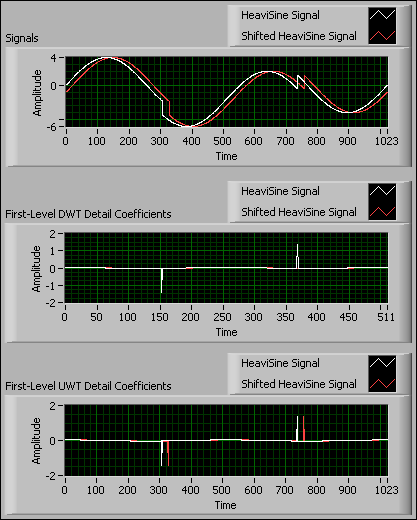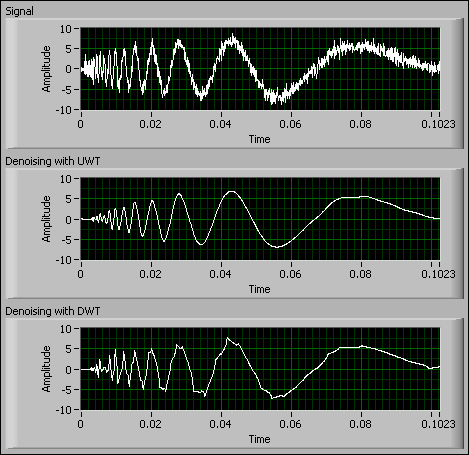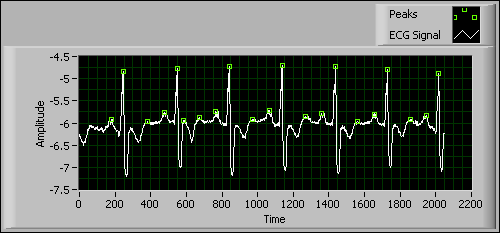# Undecimated Wavelet Transform (Advanced Signal Processing Toolkit)

LabVIEW 2014 Advanced Signal Processing Toolkit Help

Edition Date: June 2014

Part Number: 372656C-01

»View Product InfoDownload Help (Windows Only)

Unlike the discrete wavelet transform (DWT), which downsamples the approximation coefficients and detail coefficients at each decomposition level, the undecimated wavelet transform (UWT) does not incorporate the downsampling operations. Thus, the approximation coefficients and detail coefficients at each level are the same length as the original signal. The UWT upsamples the coefficients of the lowpass and highpass filters at each level. The upsampling operation is equivalent to dilating wavelets. The resolution of the UWT coefficients decreases with increasing levels of decomposition.

Use the WA Undecimated Wavelet Transform VI and the WA Inverse Undecimated Wavelet Transform VI to decompose and reconstruct 1D or 2D signals.

By comparing the UWT with the DWT, the UWT has some unique features.

## Translation-Invariant Property

Unlike the DWT, the UWT has the translation-invariant, or shift-invariant, property. If two signals are shifted versions of each other, the UWT results for the two signals also are shifted versions of each other. The translation-invariant property is important in feature-extraction applications.

The following figure shows an example that detects discontinuities in the HeaviSine signal with both the DWT and the UWT.You can use the first-level detail coefficients of either the DWT or the UWT to detect the discontinuities in the HeaviSine signal by locating the peaks in the coefficients. However, if the HeaviSine signal is shifted by 21 samples, all of the first-level DWT detail coefficients become very small. Therefore, you cannot use the first-level DWT detail coefficients to detect the discontinuities in the shifted HeaviSine signal. Because of the translation-invariant property of the UWT, you can use the first-level UWT detail coefficients to detect the discontinuities of the shifted HeaviSine Signal. The first-level UWT detail coefficients of the shifted HeaviSine Signal are simply the shifted version of the first-level UWT detail coefficients of the original HeaviSine signal.

Use the WA Get Coefficients of Undecimated Wavelet Transform VI to get the UWT coefficients you compute from the WA Undecimated Wavelet Transform VI and to return the coefficient type, such as the approximation coefficients or the detail coefficients, at a specific coefficient level. Use the WA Set Coefficients of Undecimated Wavelet Transform VI to set the coefficients you obtain from the WA Get Coefficients of Undecimated Wavelet Transform VI.

## Better Denoising Capability

Denoising with the UWT also is shift-invariant. The denoising result of the UWT has a better balance between smoothness and accuracy than the DWT. The DWT-based method is more computationally efficient than the UWT-based method. However, you cannot achieve both smoothness and accuracy with the DWT-based denoising method.

Use the Wavelet Denoise Express VI or the WA Denoise VI to reduce noise in 1D signals with both the UWT-based and DWT-based methods. The UWT-based method supports both real and complex signals. The DWT-based method supports only real signals. You also can use the WA Denoise VI to reduce noise in 2D signals with the UWT-based method.

The denoising procedure in the Wavelet Denoise Express VI and the WA Denoise VI involves the following steps:

1. Applies the DWT or the UWT to noise-contaminated signals to obtain the DWT coefficients or the UWT coefficients. The noise in signals usually corresponds to the coefficients with small values.
2. Selects an appropriate threshold for the DWT coefficients or the UWT coefficients to set the coefficients with small values to zero. The Wavelet Denoise Express VI and the WA Denoise VI provide methods that automatically select the thresholds. The bound of noise reduction with these methods is 3 dB. To achieve better denoising performance for a signal, you can select an appropriate threshold manually by specifying the user defined thresholds parameter of the WA Denoise VI.
3. Reconstructs the signal with the inverse DWT or the inverse UWT.

The following figure shows the denoising results of a noisy Doppler signal with both the DWT-based method and the UWT-based method. Both methods use the level-5 wavelet transform and the soft threshold.In the previous figure, you can see that the UWT outperforms the DWT in signal denoising because the denoised signal in the Denoising with UWT graph is smoother.

Refer to the Denoise - 1D Real Signal VI in the labview\examples\Wavelet Analysis\WAGettingStarted directory for an example of DWT-based and UWT-based methods for denoising a 1D real signal.

Refer to the Denoise - 1D Complex Signal VI in the labview\examples\Wavelet Analysis\WAGettingStarted directory for an example of DWT-based and UWT-based methods for denoising a 1D complex signal.

Refer to the Denoise - Image VI in the labview\examples\Wavelet Analysis\WAGettingStarted directory for an example of DWT-based and UWT-based methods for denoising a 2D signal.

## Better Peak Detection Capability

Peaks often imply important information about a signal. You can use the UWT to identify the peaks in a noise-contaminated signal.

The UWT-based peak detection method is more robust and less sensitive to noise than the DWT-based method, because the UWT-based method involves finding zero-crossings in the multiscale UWT coefficients. The UWT-based method first finds zero-crossings among the coefficients with coarse resolution and then finds zero-crossings among the coefficients with finer resolution. Finding zero-crossings among the coefficients with coarse resolution enables you to remove noise from a signal efficiently. Finding zero-crossings among the coefficients with finer resolution improves the precision with which you can find peak locations.

The WA Multiscale Peak Detection VI and the WA Online Multiscale Peak Detection VI use the UWT-based method. These VIs detect peaks in offline and online signals. You can use these VIs in the following ways:

• Use the WA Multiscale Peak Detection VI once for an offline signal
• Use the WA Online Multiscale Peak Detection VI continuously for a block of signals
• Use the WA Online Multiscale Peak Detection VI continuously for signals from streaming data sources

The following figure shows an example that uses the WA Online Multiscale Peak Detection VI to detect peaks in an electrocardiogram (ECG) signal. The UWT-based method locates the peaks of the ECG signal accurately, regardless of whether the peaks are sharp or rounded.Refer to the ECG Heart Rate Monitor (Online) VI in the labview\examples\Wavelet Analysis\WAApplications directory for an example of how to use the WA Online Multiscale Peak Detection VI to detect peaks in an ECG signal.

Refer to the ECG QRS Complex Detection VI in the labview\examples\Wavelet Analysis\WAApplications directory for an example of wavelet-based peak detection in biomedical engineering.

Refer to the Peak Detection (Wavelet vs. Normal) VI in the labview\examples\Wavelet Analysis\WAGettingStarted directory for an example that compares the wavelet transform-based peak detection method with the traditional curve fitting-based method.